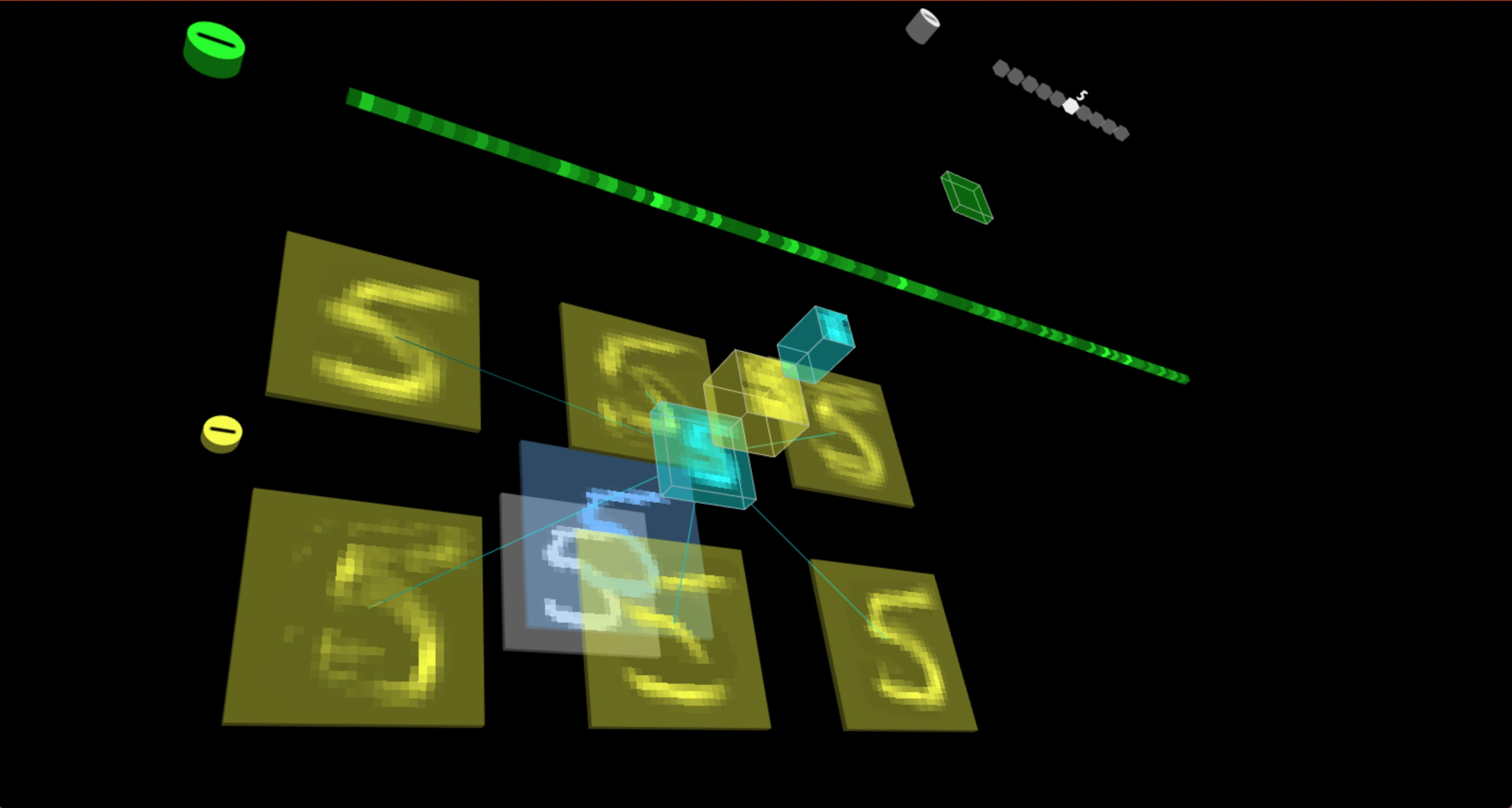TensorSpace.js
Getting Start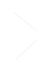Basic ConceptsTensorSpace ConverterModel PreprocessingModelsLayersMerge Function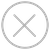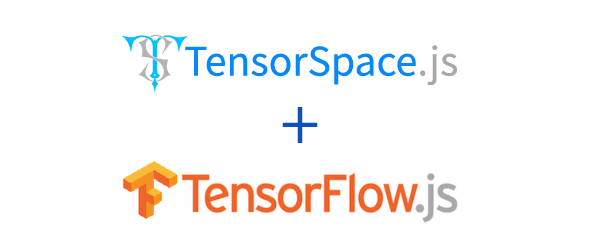Visualize pre-trained TensorFlow.js model using TensorSpace and TensorSpace-Converter
Introduction
In the following chapter, we will introduce the usage and workflow of visualizing TensorFlow.js model using TensorSpace and TensorSpace-Converter. In this tutorial, we will convert a TensorFlow.js model with TensorSpace-Converter and visualize the converted model with TensorSpace.
This example uses LeNet trained with MNIST dataset. If you do not have any existed model in hands, you can use this script to train a LeNet TensorFlow.js model. We also provide pre-trained LeNet model for this example.
Sample Files
The sample files that are used in this tutorial are listed below:
filter_center_focus pre-trained TensorFlow.js model (mnist.json and mnist.weight.bin)
Preprocess
First we will use TensorSpace-Converter to preprocess pre-trained TensorFlow.js model:
``````\$ tensorspace_converter \
--input_model_from="tfjs" \
./rawModel/mnist.json \
./convertedModel/``````
wb_sunnyNote:
• filter_center_focus Set input_model_from to be tfjs.
• filter_center_focus A pre-trained model built by TensorFlow.js, may have a topology file xxx.json and a weights file xxx.weight.bin, the two files should be put in the same folder and set topology file's path to positional argument input_path.
• filter_center_focus Get out the layer names of model, and set to output_layer_names like Fig. 1.
• filter_center_focus TensorSpace-Converter will generate preprocessed model into convertedModel folder, for tutorial propose, we have already generated a model which can be found in this folder.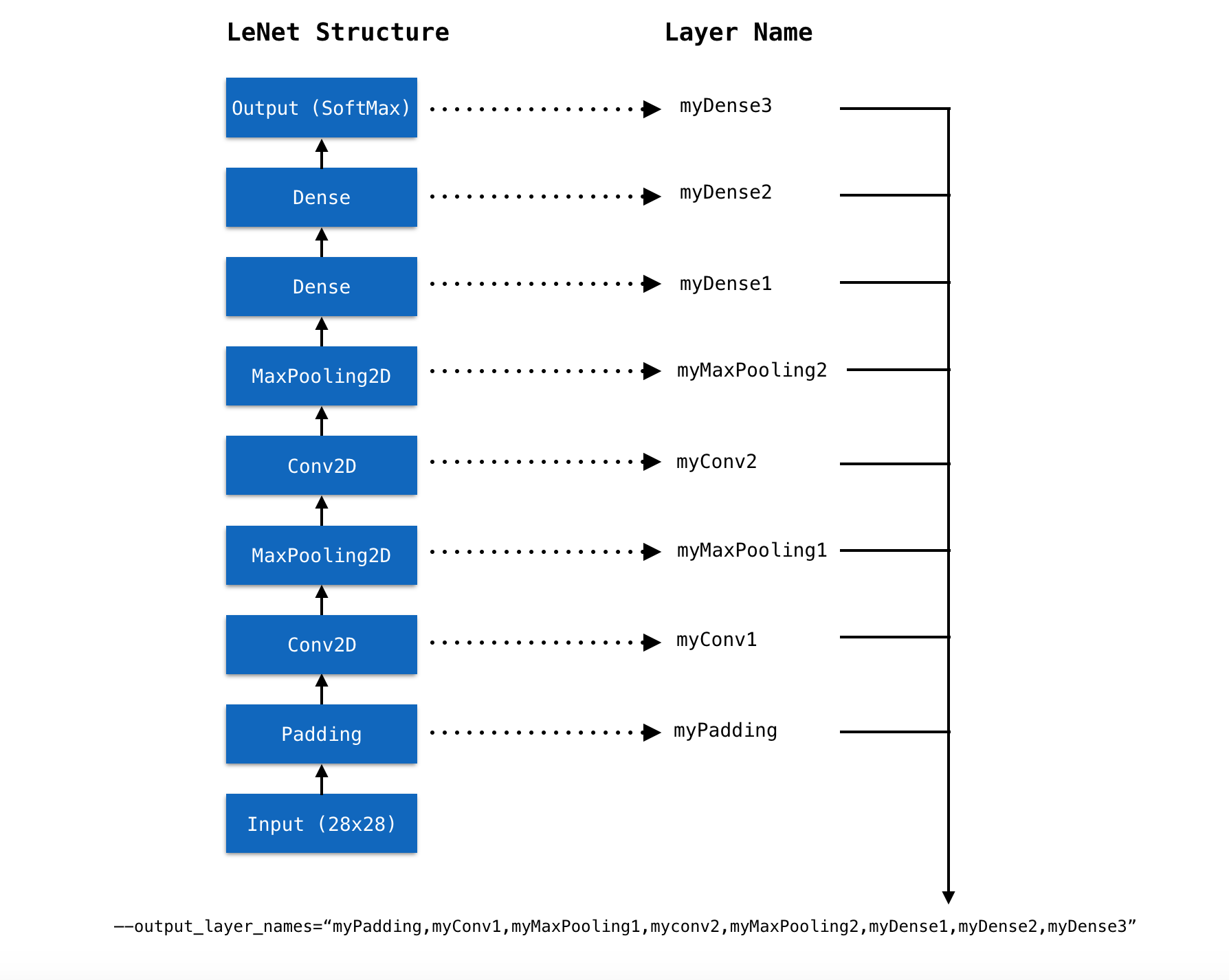Fig. 1 - Set TensorFlow.js layer names to output_layer_names
After converting, we shall have the following preprocessed model:Fig. 2 - Preprocessed TensorFlow.js model
wb_sunnyNote:
• filter_center_focus There are two types of files created:
• flare .json is for the model structure
• flare .bin is the trained weights
Then Apply TensorSpace API to construct visualization model.
``````let model = new TSP.models.Sequential( modelContainer );

``````model.load( {